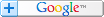ALL YOUR EXCEL & SOFTWARE NEEDSFREE Excel STUFF Advanced SearchPRODUCTS Development Contact UsExcel Online# 4 Criteria VLOOKUP In Excel

Back to: Excel Custom Function/Formulas

This Custom Function can use the 1st 4 Columns of a table as matching criteria and return the nth column row to the right of the matching row range.

```Function Four_Con_Vlookup(Table_Range As Range, Return_Col As Long, Col1_Fnd, Col2_Fnd, Col3_Fnd, Col4_Fnd)

''EXCEL 2003 OR ABOVE

''WRITTEN BY OZGRID.COM

Dim rCheck As Range, bFound As Boolean, lLoop As Long

On Error Resume Next

Set rCheck = Table_Range.Columns(1).Cells(1, 1)

With WorksheetFunction

For lLoop = 1 To .CountIf(Table_Range.Columns(1), Col1_Fnd)

Set rCheck = Table_Range.Columns(1).Find(Col1_Fnd, rCheck, xlValues, xlWhole, xlNext, xlRows, False)

If UCase(rCheck(1, 2)) = UCase(Col2_Fnd) And _

UCase(rCheck(1, 3)) = UCase(Col3_Fnd) And _

UCase(rCheck(1, 4)) = UCase(Col4_Fnd) Then

bFound = True

Exit For

End If

Next lLoop

End With

If bFound = True Then

Four_Con_Vlookup = rCheck(1, Return_Col)

Else

Four_Con_Vlookup = "#N/A"

End If

End Function```

OR

```Function Four_Con_Vlookup(Table_Range As Range, Return_Col As Long, Col1_Fnd, Col2_Fnd, Col3_Fnd, Col4_Fnd)

''PRE EXCEL 2003

''WRITTEN BY OZGRID.COM

Dim rCheck As Range, bFound As Boolean, lLoop As Long, lRow As Long

On Error Resume Next

Set rCheck = Table_Range.Columns(1).Cells(1, 1)

With WorksheetFunction

For lLoop = 1 To .CountIf(Table_Range.Columns(1), Col1_Fnd)

lRow = .Match(Col1_Fnd, Table_Range.Columns(1).Range("A" & lRow + 1 & ":A65536"), 0) + lRow

Set rCheck = Table_Range.Columns(1).Range("A" & lRow)

If UCase(rCheck(1, 2)) = UCase(Col2_Fnd) And _

UCase(rCheck(1, 3)) = UCase(Col3_Fnd) And _

UCase(rCheck(1, 4)) = UCase(Col4_Fnd) Then

bFound = True

Exit For

End If

Next lLoop

End With

If bFound = True Then

Four_Con_Vlookup = rCheck(1, Return_Col)

Else

Four_Con_Vlookup = "#N/A"

End If

End Function```

Use like;
=Four_Con_Vlookup(\$A\$1:\$H\$20,6,"Apr",4,"Thu","Larry")
in any cell other than \$A\$1:\$H\$20

Where \$A\$1:\$H\$20 is the table range

6 is the nth column to return the matching row from.

"Apr",4,"Thu" and "Larry" are the 4 conditions to match is the 1st 4 Column

Excel Dashboard Reports & Excel Dashboard Charts 50% Off Become an ExcelUser Affiliate & Earn Money

Special! Free Choice of Complete Excel Training Course OR Excel Add-ins Collection on all purchases totaling over \$64.00. ALL purchases totaling over \$150.00 gets you BOTH! Purchases MUST be made via this site. Send payment proof to [email protected] 31 days after purchase date.Search Tips FREE Excel Help